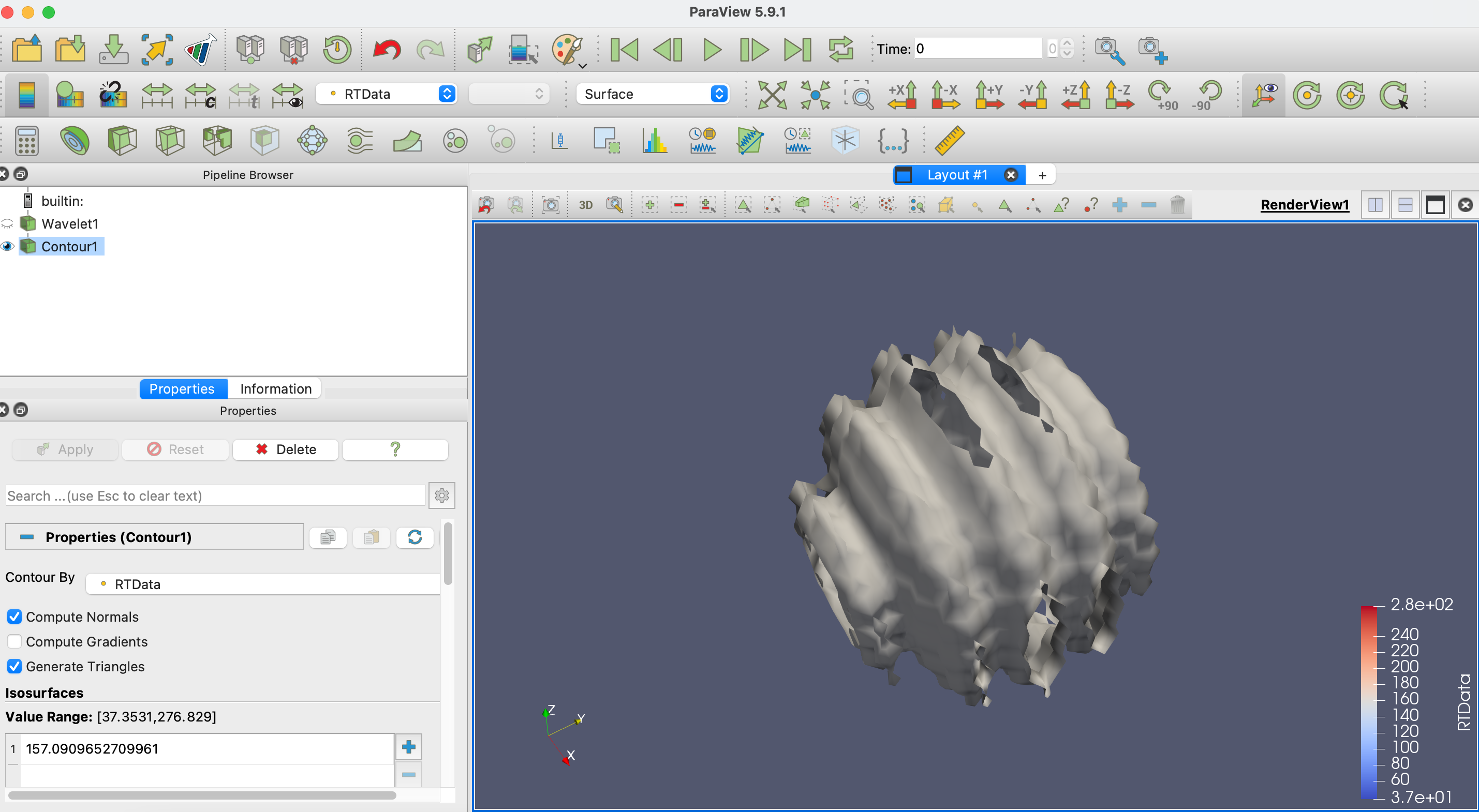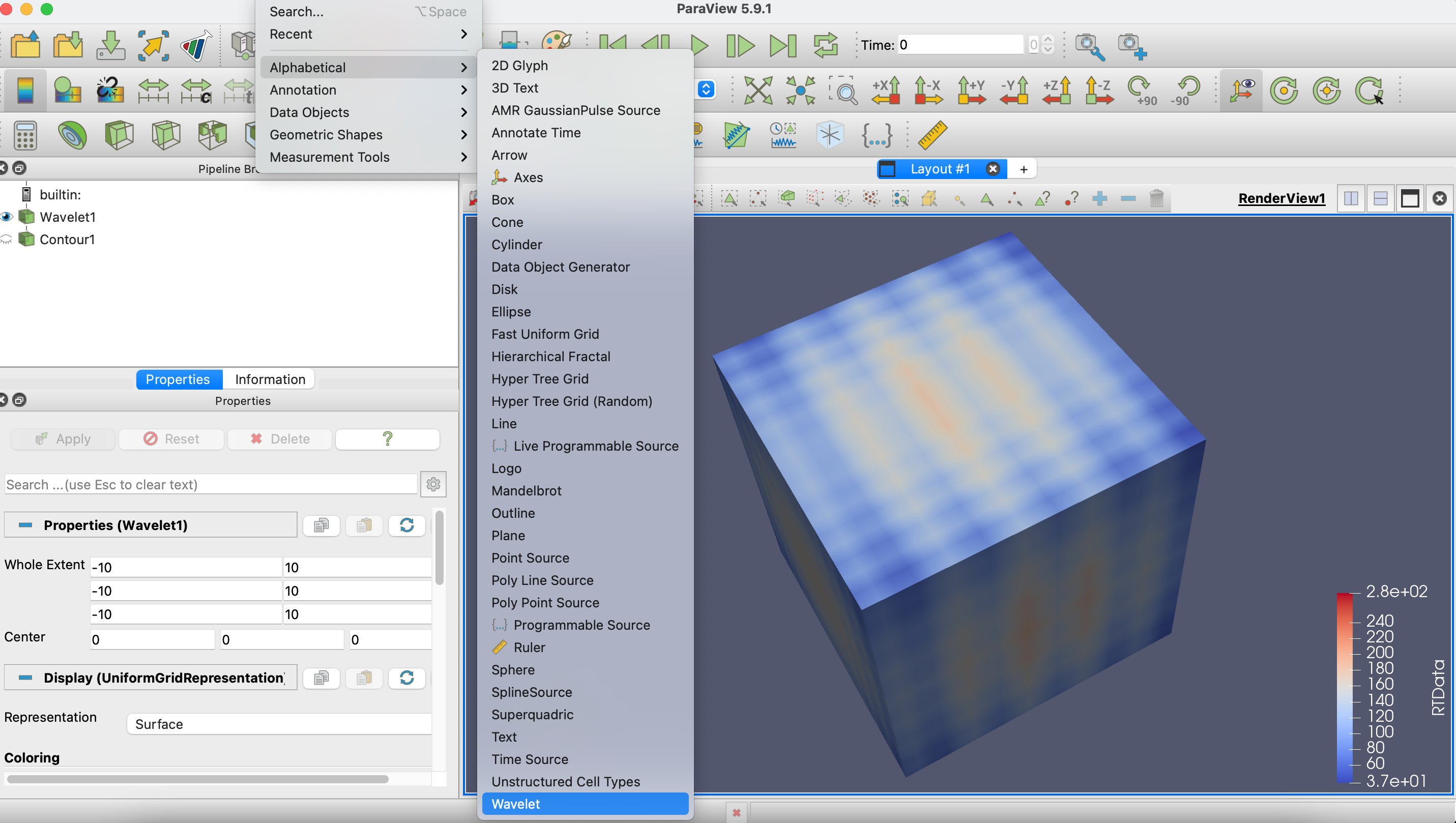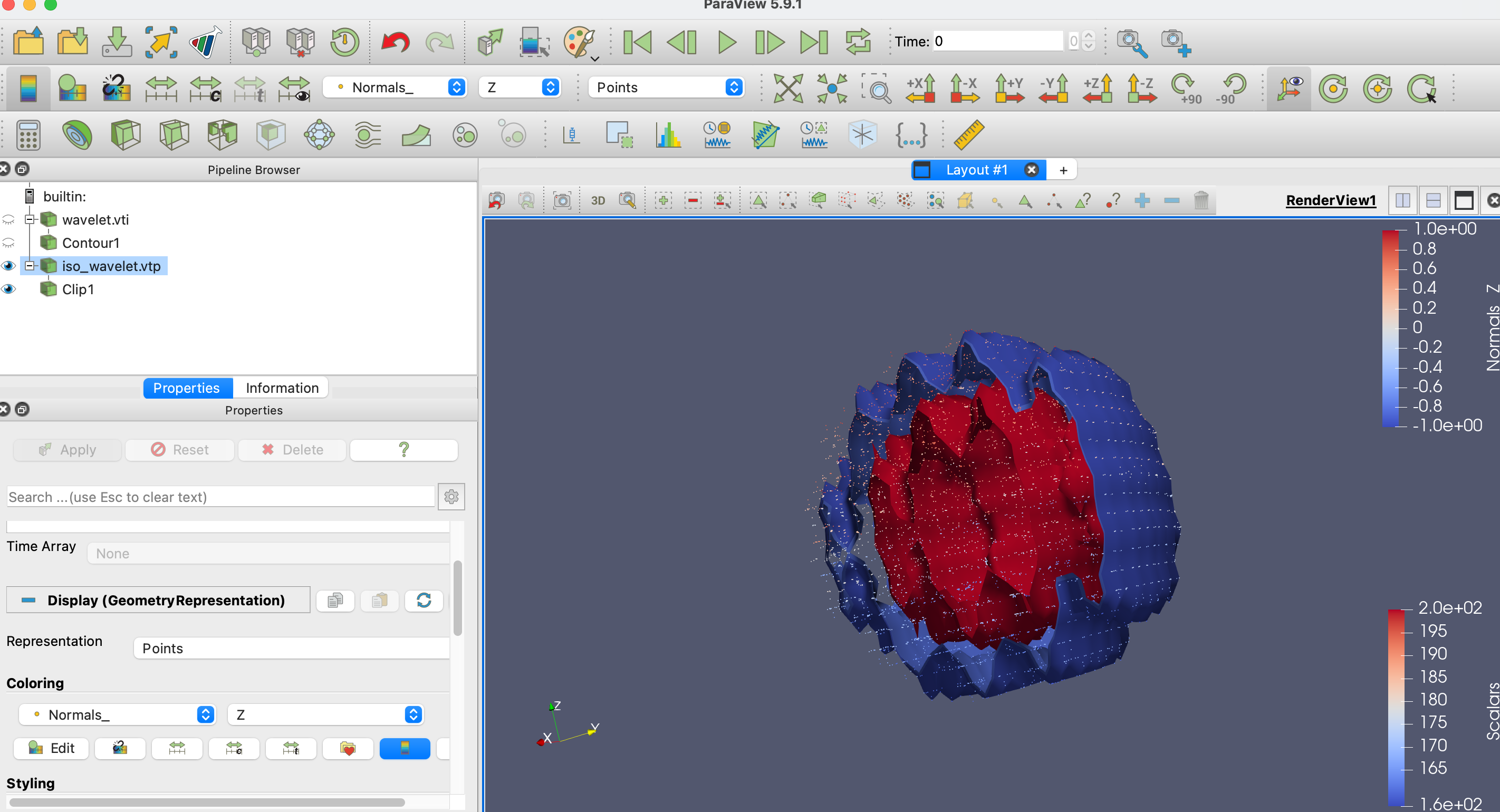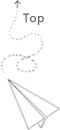# Different Levels of Understanding Marching Cube

This is the scripts for this video

Isosurface might be one of the most common algorithm for analysing the scientific data. In this video, we try to decompose it into multiple levels to explain how to understand and use the marching cube algorithm to achieve the isosurface step by step. Specifically, we will go through every steps of the marching cube algorithm and we will also use the VTK as the implementation library to understand the details of the marching cube algorithm.

### Level 1

The goal of the first level is simple, we just need to know how to use marching cube algorithm from the perspective of programming. We try to figure out what it can do, what is the input and output of the algorithm.

We first show that how to do the isocountor operation in the Paraview, which is a desktop software based on the VTK.Here, We try to generate a data source from the paraview such as wavelet1 shown in the figure above, and then click the contour button, then click the apply, by this way, the countour surface with specific value is created, which is shown in the figure.

If we try to do the isosurface operation by ourself with the cpp code, we may first save the wavelet data into the disk firstly. You may get the sample file here

We then need to load the image file and generate the vtkImageData in cpp program, please check the video below to see how to read the vtk image file by xml reader if you are not familiar with the VTK data read and write operation.

If you do not familiar with the process to build the program that uses VTK library based on cmake, you may check all kinds of exmaples here, we also list the link below this vedio

With this example code, we can get the image data and printout all its array. We could see that there is a field value associated with every data point. So, the input data of the maching cube is the typical image data, and the field data is attached to the image point.

The next step is try to connect the data with the marching cube filter and get the rendered figures.

The code example listed here insert several iso values and then output a polygonal data object that describes these values. We may also render the existing vtk object direactly based on online example, here we just try to write out the vtk polygonal data by the vtk file and render it on the paraview. In summary, this example shows that we input the vtk image data and the filter returns the vtk polygonal data.The above code and associated image data can be found here and we also list the link below this video.

### Level 2

From the example shown in Level 1, we have some ideas about how to use the VTK library to execute the marching cube algorithm, the goal of the level 2 is try to understand key ideas behind this algorithm and how it is implemented. We use two references in this video, the first is the vtk document online about the marching cube algorithm here, the second reference is the original paper that describes the marching cube algorithm: Lorensen W E, Cline H E. Marching cubes: A high resolution 3D surface construction algorithm[J]. ACM siggraph computer graphics, 1987, 21(4): 163-169.

Let’s look at some core ideas behind this algorithm together.

We use two questions as the outline for the marching cube method:

(1) How to map the original mesh (which is a grid) into the format that we can compare with the threshold value?
(2) How to map the compared results into the new data representation, namely the polygonal data?

The input contains the regular grid, such as image. and a threshold value that try to extract. The output is the unstructured grid, such as the polygonal data.

For the convenience of the explanation, we use the 2d grid as the example here, the grid data can be both in 2d or 3d in practice.

As shown in the Figure (6.4 of the VTK book). The image data is constructed by structured points and edge, we select out one cell from them, In this example, the scalar is binded with each vertex of the grid. If we assume evey pixels in this cell contains a particular scalar value, but we only know the exact scarlar value at four coner points. How could we draw a line to split it?

The naive idea is to use the interpolation, we can find the particular point among the edge and then draw a line as the approximation. Such as the example shown in case 1 and case 8 of the Figure 6-5. The position on the edge can be selected based on interpolation.

This is simple idea to map the scalar value into the topology representation. If our 2d face contains multiple small cells, we just need to go through each cell one by one, and execute above operations, we can get a contour around the threshold value we input, we can get high granularity countour if we use more cells.

If we consider any scarlar value and a customized countour value we want to extract, it is either larger than the contour value or smaller then contour value. If we use the topoligical relationship to understand it, it can be either outside the contour face or inside the contour face. By this way, we build a connection between the comparision of scarlar value and the topological positions. For every grid cell, there are four points, and given one threshold, there are 2^4 = 16 cases in total. The Figure 6-5 of the VTK book shows all 16 cases. The simple way is to use a digit number to represent all cases. We basically need 4 digits that represent each points. If the particular digit is 1, it means the value is larger than threshold, if it is 0, it means the value is smaller than threshold. Then we can build a case table, each combination corresponds with a case shown in Figure 6-5. If we extend it into 3d cases, there are 8 points and totally 256 cases.

If we use multiple steps to express the above process, the algorithm can be described as follows:

You may need to read the original paper to get more detials, but I believe you can understand main ideas of the marching cube algorithm till this step.

### Level 3

For the Level 3, we try to further dive into the maching cube algorithm. We try to figure it out how it is implemented. Let’s take the VTK library as an example to explain it in details. The VTK library contains different implementaions of the marching cube algorithms with different efficiency: such as vtkContour, vtkMarchingCube and vtkFlyEdge We mainly look at the vtkMarchingCube in this article.

The best way to understand the code is to run it and add some print log to see what’s going on here. This is the eample we use to understand the marching cube algorithm here, we basically copy past origianl header and source file from the VTK library and get our own version of marchingcube algorithm and compile it separately. By adding all kinds of logs, we can basically figure out the main logic of the algorithm. It is also a good practice to understand other VTK filters by this way.

We list some key points for understanding the source code of vtkMarchingCube.cxx

The extract the input data here

we have the extracted scarlar array. Altough it is an 1d array, it represent the 3D image data logically.

Dispatcher worker approach is the way to execute the for loop in the VTK, you may refer to this video about the dispatcher worker approach to do the vtk array operation. This is the place where the worker is called.

The core operation of the worker is a three layered for loop we try to go through all points and seletct different cell with each point as the started position. The s array here contains the scarlar value of each selected cell. And the pts represents the coordinates for selected voxel in 3d space.

With these key data structures, we start to look up case table and map the comparison results between the scarlar value and the threshold value into a particular topological shape.

The index of the case table is calculated here, the VTK libraray list all 256 cases here, the nonzero value represents the index of the edge that we need to intersect with.

There are at most 5 triangles for the marching cube case for the voxel, and we need 15 indexes to represent it, the last one is a termination digit. Altoght we can simplify 256 cases into the 16 cases, however, from the programming perspective, all kinds of rotation is error prone, so we use the strategy of space to time to make it more simple.

Then we go through each edge where there is intersection and calcaulte the coordinates of the intersect points based on interpolation. Then use the locator to find a particular points based on the indecies

https://github.com/Kitware/VTK/blob/master/Filters/Core/vtkMarchingCubes.cxx#L337

Then insert the newpoint into the polygonal data based on next cell function.

### Level 4 and more

The contents in level 4 are out of the topic of current video. You may need to know some optimization topics, such as calculating the gradients and consider how to merge shaps between the voxel. etc. You may even try to implement whole algorithm by your self as needed. Besides, there are more efficient marching cube implementation based on parallel primitives, such as class vtkFlyingEdges3D.

Thanks for watching, remember to subscribe our channel if the contents are helpful for you.

VTK discussion about the case table